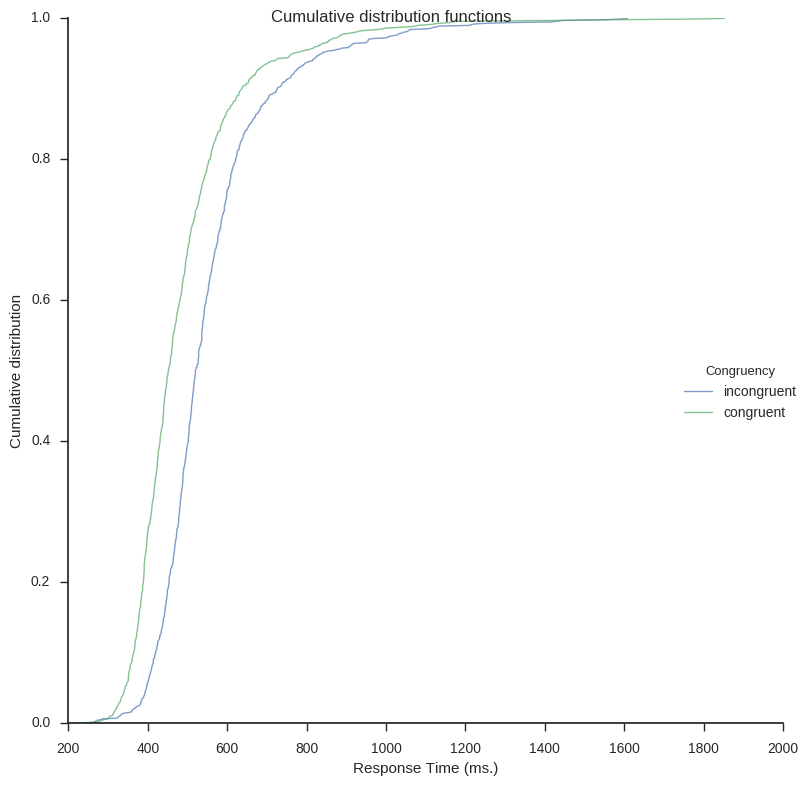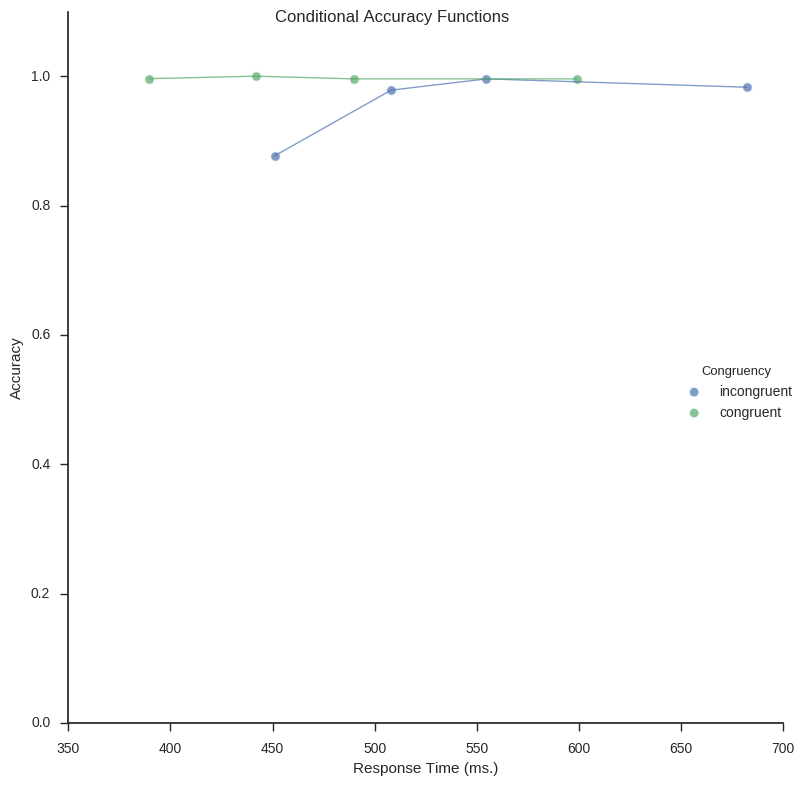Inspired by my post for the JEPS Bulletin (Python programming in Psychology), where I try to show how Python can be used from collecting to analyzing and visualizing data, I have started to learn more data exploring techniques for Psychology experiments (e.g., response time and accuracy). Here are some methods, using Python, for visualization of distributed data that I have learned; kernel density estimation, cumulative distribution functions, delta plots, and conditional accuracy functions. These graphing methods let you explore your data in a way just looking at averages will not (e.g., Balota & Yap, 2011).

## Required Python packages

I used the following Python packages; Pandas for data storing/manipulation, NumPy for some calculations, Seaborn for most of the plotting, and Matplotlib for some tweaking of the plots. Any script using these functions should import them:

## Kernel Density Estimation

The first plot is the easiest to create using Python; visualizing the kernel density estimation. It can be done using the Seaborn package only. kde_plot takes the arguments df Note, in the beginning of the function I set the style to white and to ticks. I do this because I want a white background and ticks on the axes.

Using the function above you can basically plot as many conditions as you like (however, but with to many conditions, the plot will probably be cluttered). I use some response time data from a Flanker task:

## Cumulative Distribution Functions

Next out is to plot the cumulative distribution functions (CDF). In the first function CDFs for each condition will be calculated. It takes the arguments df (a Pandas dataframe), a list of the conditions (i.e., conditions).

Next is the plot function (cdf_plot). The function takes a Pandas a dataframe (created with the function above) as argument as well as save_file and legend.

Here is how to create the plot on the same Flanker task data as above:## Delta Plots

In Psychological research Delta plots (DPs) can be used to visualize and compare response time (RT) quantiles obtained under two experimental conditions. DPs enable examination whether the experimental manipulation has a larger effect on the relatively fast responses or on the relatively slow ones (e.g., Speckman, Rouder, Morey, & Pratte, 2008).

In the following script I have created two functions; calc_delta_data and delta_plot. calc_delta_data takes a Pandas dataframe (df). Rest of the arguments you need to fill in the column names for the subject id, the dependent variable (e.g., RT), and the conditions column name. All in the string data type. The last argument should contain a list of strings of the factors in your condition.

Next function, delta_plot, takes the data returned from the calc_delta_data function to create a line graph.

The above functions are quite easy to use. First load your data (again, I use data from a Flanker task).

## Conditional Accuracy Functions

Conditional accuracy functions (CAF) is a technique that also incorporates the accuracy in the task. Creating CAFs involve binning your data (e.g., the response time and accuracy) and creating a linegraph. Briefly, CAFs can capture patterns related to speed/accuracy trade-offs (see Richard, 2014). First function,

caf_plot (the function below) uses Seaborn, again, to plot the conditional accuracy functions.

Right now, the function for calculation the Conditional Accuracy Functions can only do one condition at the time. Thus, in the code below I subset the Pandas dataframe (same old, Flanker data as in the previous examples) for incongruent and congruent conditions. The CAFs for these two subsets are then concatenated (i.e., combined to one dataframe) and plotted.Conditional Accuracy Functions (CAFs) created using Python and Seaborn

Update: I created a Jupyter notebook containing all code: Exploring distributions.

### References

Balota, D. a., & Yap, M. J. (2011). Moving Beyond the Mean in Studies of Mental Chronometry: The Power of Response Time Distributional Analyses. Current Directions in Psychological Science, 20(3), 160–166. http://doi.org/10.1177/0963721411408885

Luce, R. D. (1986). Response times: Their role in inferring elementary mental organization (No. 8). Oxford University Press on Demand.

Richard, P. (2014). The speed-accuracy tradeoff : history , physiology , methodology , and behavior. Frontiers in Neuroscience, 8(June), 1–19. http://doi.org/10.3389/fnins.2014.00150

Speckman, P. L., Rouder, J. N., Morey, R. D., & Pratte, M. S. (2008). Delta Plots and Coherent Distribution Ordering. The American Statistician, 62(3), 262–266. http://doi.org/10.1198/000313008X333493

1.Klaas Bombeke

Hi, thank you for the great tutorial on delta plots, it was very helpful! Am I correctly assuming that index “rt” should be “dv” (lines 24&25) and “n_subjects” should be “subjects” (line 15) in order to use it straight away with my own data? Thank you.

•Erik Marsja

Hi Klaas,

Glad you found it helpful and yes, you are correct. I will update and correct the code example!

Erik

This site uses Akismet to reduce spam. Learn how your comment data is processed.